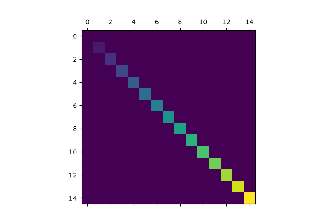# matplotlib.pyplot.matshow#

matplotlib.pyplot.matshow(A, fignum=None, **kwargs)[source]#

Display an array as a matrix in a new figure window.

The origin is set at the upper left hand corner and rows (first dimension of the array) are displayed horizontally. The aspect ratio of the figure window is that of the array, unless this would make an excessively short or narrow figure.

Tick labels for the xaxis are placed on top.

Parameters:
A2D array-like

The matrix to be displayed.

fignumNone or int

If None, create a new, appropriately sized figure window.

If 0, use the current Axes (creating one if there is none, without ever adjusting the figure size).

Otherwise, create a new Axes on the figure with the given number (creating it at the appropriate size if it does not exist, but not adjusting the figure size otherwise). Note that this will be drawn on top of any preexisting Axes on the figure.

Returns:
`AxesImage`
Other Parameters:
**kwargs`imshow` arguments

## Examples using `matplotlib.pyplot.matshow`#Visualize matrices with matshow

Visualize matrices with matshow